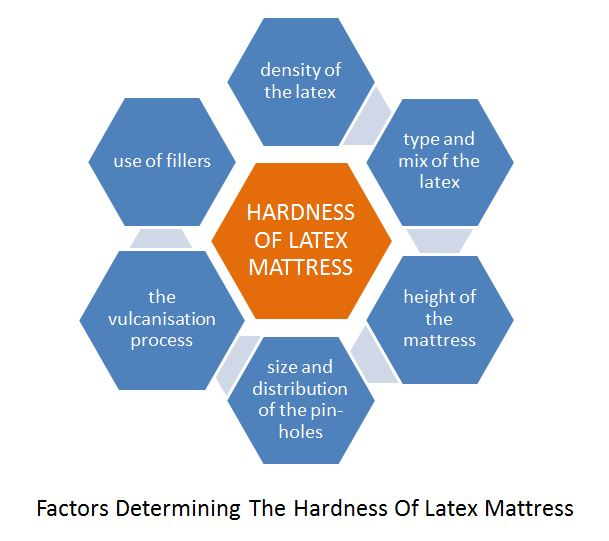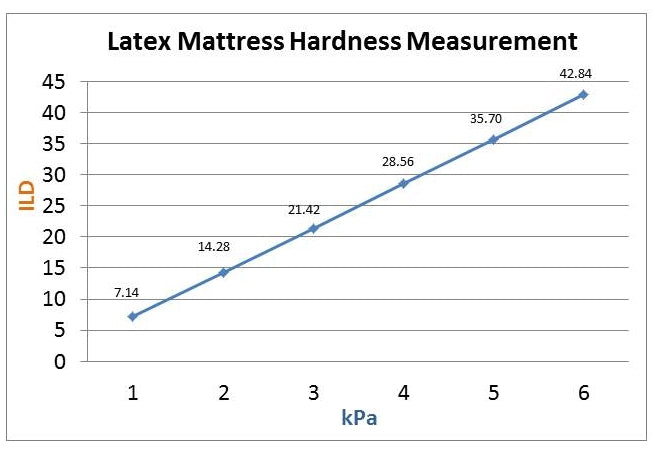COMFORT MEETS NATURE

Density, Hardness, Resilience & Weight Of Latex Mattresses

Last week, I received a call from a customer enquiring about the density and weight of our latex mattresses. He was only interested in natural latex mattresses. He didn’t want to consider blended latex mattresses nor organic latex mattresses. He has done some research online and was aware of using density to determine the firmness (firm, medium or soft) of the mattress. When I replied that the densities our latex mattresses vary from 65 to 85 kg/m³ depending on the brands and models. He seemed a little confused and wanted to find out which range of our mattresses has an ILD closed to 36.

In this blog post, I would like to discuss about the difference between density and hardness of latex mattresses and the various ways of measuring them. We will also take a look at another factor, resilience, which determines the elasticity of mattress.

1. What is density?
Density of our latex mattresses is usually expressed by mass per unit volume. It is a value measured by kg / m³ and is derived through the weighing of a standardized sample (ISO 845). The densities of our latex mattresses vary between 65 to 85 kg/m³. The density simply indicates the quantity (kg) of material used in a specific area (m³). Therefore, the firmness of the mattress is correlated to density - the more material is used, the firmer the mattress is.

2. What is hardness?
When you press on a mattress, you will feel some form of resistance. This resistance is known as the hardness of a mattress. So when you press down on a latex mattress, you will feel the latex core pushing up. The more pressure you exert on the latex core, the more it pushes up and that indicates the hardness of the mattress.

Hardness is the resistance against exerted pressure. Hardness and density (mass per unit volume) of a latex mattress are related or connected to each other. Over a long period of usage, as the density of a latex mattress decreases due to repeated loading or pressure, the mattress will also lose its hardness.

There are several important factors that will determine the hardness of a latex mattress. They are;
• density of the latex
• type and mix of the latex
• height of the mattress
• size and distribution of the pin-holes
• the vulcanisation process
• use of fillers2a. How to measure it?

One method to measure hardness of a mattress is by using the standard ISO 2439 method, which states that the ILD-hardness (Indentation Load Deflection) measures the pressure that is required to load the mattress core till 25%, 40% or 60% compared to its original thickness with a circular plate of 50 square inches or 322 cm².

The other method to measure hardness is by using the CLD-hardness (Compression Load Deflection) which is in accordance to the standard ISO 3386, Latexco Belgium, where most of our European Bedding latex cores are manufactured, uses this method which is the counter pressure (= force per surface) in Pascal when 25% of the mattress core is pressed in with the same circular plate. Take note that 1 kPa (kilopascal) equals 10 g/cm² (gram per square cm).,Measuring & Testing The Density & Hardness Of Latex Mattress

3. How to express this pressure?

Pressure is expressed in Kilo-Pascal ( kPa ).
1 Pascal = 1 Newton ( a measure of Force ) / m²
1 kPa = 1000 Pascal and 1 m² = 10,000 cm²; so 1 kPa = 0.1 N/cm²
So if you press down with the circle stamp of 322cm², calculation is as follows :
1 kPa = 0.1 N/cm² x 322cm² = 32.2 Newton (Only in case of pressing with a 322 cm² stamp!)

In general we can say a
• soft mattress : +/- 3.5 kPa
• medium mattress : +/- 4 kPa
• firm mattress : +/- 4.5 kPa
4. What is the difference between ILD and kPa?

ILD or Indentation Load Deflection is one measure of load bearing and is expressed in pounds per 50 square inches at a given percentage deflection of the foam (normally 25%).

To obtain the value, a 50 square inch circular plate is pushed into the foam surface, stopping at a given deflection and reading the load.

For example a 25 percent ILD of 30 means that it takes 30 pounds load to compress a 4 inch thick piece of foam to a 3 inch thickness. So, an ILD standard 34 and 37 (pounds) equals the hardness of 4.8 and 5.2 kPa, given 0.4536 kilogram per pound and 322.58 square centimeters for the
50 square inch stamp.

Formula : 1 ILD = 0.14 Kpa or 1 Kpa = 7.14 ILD

ILD is used in countries which use the old English system. However, kPa, the metric system - an internationally agreed system of measurement, is now used in almost every country in the world.5. What is resilience?

Resilience for mattresses refers normally refers to elasticity. It is measured by the "ball-rebound"-test (ASTM D3574). In this test, a steel ball is dropped off from a certain height on the material and  the rebound is then measured in percentage compared to the predetermined height. The resilience or elasticity of regular Polyurethane (PU) foams is between 40% and 50%. High Resilience (HR) polyurethane foams have an elasticity of 50% to 60% and latex foams of 60% to 70%. Slow recovery foams have zero elasticity!

6. How to calculate the weight of a mattress?

Using the length, width, height (thickness) and density of the mattress, we can easily calculate the weight of the mattress. For example, for our EB Back Care Perfect King Size latex mattress, the latex core length is 2m, the width is 1.8m and the height is 0.18m. Let’s calculate the weight of;
• firm mattress – 2 x 1.8 x 0.18 x 75 = 48.6kg
• medium mattress – 2 x 1.8 x 0.18 x 70 = 45.36kg
• soft mattress – 2 x 1.8 x 0.18 x 65 = 42.12kg